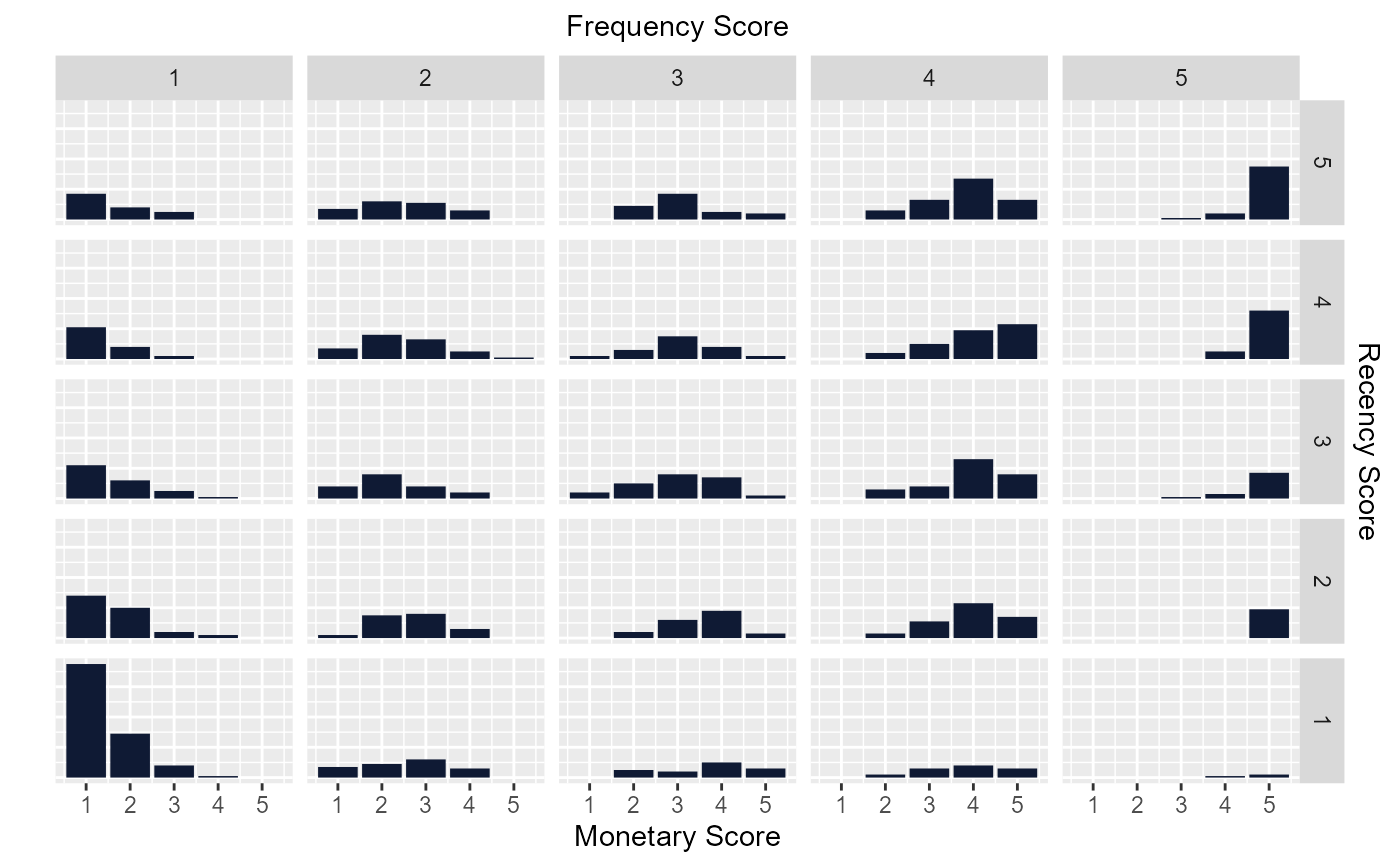Examine the distribution of monetary scores for the different combinations of frequency and recency scores.

## Usage

rfm_plot_bar_chart(
rfm_table,
bar_color = NULL,
xaxis_label = NULL,
sec_xaxis_label = NULL,
yaxis_label = NULL,
sec_yaxis_label = NULL,
print_plot = TRUE
)

## Arguments

rfm_table

An object of class rfm_table.

bar_color

Color of the bars.

xaxis_label

X axis label.

sec_xaxis_label

Secondary x axis label.

yaxis_label

Y axis label.

sec_yaxis_label

Secondary y axis label.

print_plot

logical; if TRUE, prints the plot else returns a plot object.

Bar chart.

## Deprecated Functions

rfm_bar_chart() has been deprecated and will be made defunct. It has been provided for compatibility with older versions only, and will be made defunct at the next release.

Instead use the replacement function rfm_plot_bar_chart().

## Examples

# using transaction data
analysis_date <- as.Date('2006-12-31')
rfm_order <- rfm_table_order(rfm_data_orders, customer_id, order_date,
revenue, analysis_date)

# bar chart
rfm_plot_bar_chart(rfm_order)